# Adding Fun to First-Year Computer Programming Classes with MATLAB, Arduino Microcontrollers, and Model Trains

By Lowell Toms and Dustin West, Ohio State University

Ohio State University’s First-Year Engineering course sequence is designed to give incoming students insight into most of the engineering disciplines offered at the university. The challenge is to keep the students interested and prevent that first year from turning into a painful slog through the core science and math courses. How do we solve that challenge? We let the students play with train sets while teaching them basic programming skills using MATLAB®.

The 1700 students who take ENGR 1181: Fundamentals of Engineering I each year not only learn engineering concepts and the basics of computer programming in MATLAB; they also complete a final project in which they develop a controller for an N scale train set using MATLAB and an Arduino® microcontroller. This project enables students to demonstrate what they’ve learned. It also gives them their first opportunity to experience the joy of engineering by seeing the results of their efforts come to life in the lab. We frequently see students jumping and cheering as they watch their control algorithms work for the first time.

## Learning Programming with MATLAB

Before they tackle the train project, students learn measurement, graphing, data analysis, and other core engineering concepts in parallel with the basics of programming. Lab assignments in MATLAB reinforce the programming lessons, which cover arrays, strings, loops, logical expressions, conditionals, and other constructs.

Most students come to the course with little or no programming experience. We have found that MATLAB is an excellent first language to learn. MATLAB is more interactive than C and similar languages, there is no need to compile, and there are far fewer low-level details for the students to master. Once they understand basic programming concepts in MATLAB, students learn to apply them in other languages quite rapidly. MATLAB is also ideal for ENGR 1181 because it is used across all engineering disciplines at Ohio State.

## Controlling Trains with MATLAB and Arduino Hardware

Students begin the train project during the 10th week of the course. Their goal is to develop a MATLAB controller that works with an Arduino microcontroller to interact with a train as it travels around a circular track. Break-beam sensors separate the track into a “city” section and a “country” section (Figure 1). As the train enters the city, the MATLAB code must reduce the train speed, start the road crossing flashers, and then drop the crossing gate after a one-second delay. When the train leaves the city and enters the country, the MATLAB code must increase the train speed, turn off the flashers, and raise the gate.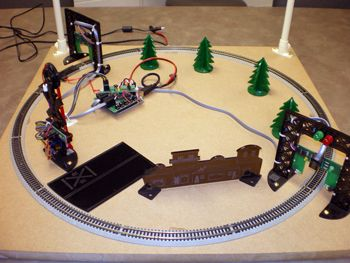Figure 1. N scale train setup in the lab.

Working in groups of four, the students use MATLAB and the MATLAB Support Package for Arduino Hardware to write a program that communicates with an Arduino Uno via a serial port. The program checks the status of the break-beam sensors via the Arduino’s digital input, and uses the Arduino’s digital output to flash the LEDs and operate a servo motor for raising and lowering the gate.

Over the course of three lab sessions, the students implement their MATLAB control algorithms. These typically consist of an endless polling loop with conditionals to check the status of sensors and logic to manage the servos and LEDs.

For many students, the most challenging aspect of the project is implementing a nonblocking delay, needed to flash the LEDs and lower the gate after one second. Students discover that they cannot use the MATLAB `pause()` function because the algorithm may miss sensor input while the pause is executing. Instead, they learn to use MATLAB `tic()` and `toc()` functions to time the delay without interrupting the polling loop (Figure 2).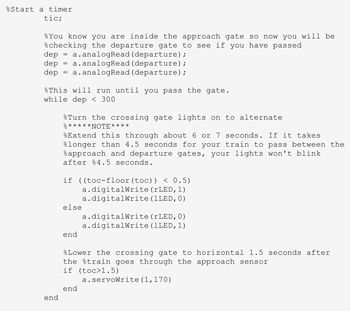Figure 2. Student code using tic() and toc() to implement a nonblocking delay.

## Building a Simulator for Debugging and Independent Work

Scheduling lab time for 1700 students was a significant challenge. Our lab has room for 18 tables, each capable of holding one train setup. With four-member teams, we could accommodate just 72 students at a time. Long waits for lab time were made even longer because students had to do all their debugging on an actual train set in the lab. Another concern was that one member of each team—usually someone with prior programming experience—tended to do most of the work while the other three watched.

To address these challenges, two graduate students developed a train simulator in MATLAB that emulates the train setups in the lab (Figure 3). The simulator uses a MATLAB figure window to draw the track, train, break-beam sensors, and gate. Students can use the simulator to debug their control algorithm code and then use the same code on the actual train set. Teams then spend lab time optimizing their algorithms instead of debugging, greatly reducing the amount of time each team needs with the actual trains. Further, we can now give assignments for students to complete independently using the simulator, ensuring that no single programmer does all the work.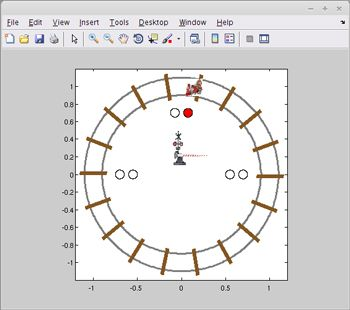Figure 3. The MATLAB train set simulator.

## MATLAB in the Second Semester and Beyond

Engineering students continue to use MATLAB in ENGR 1182: Fundamentals of Engineering 2. In this course, the students must design and build an advanced energy vehicle that uses as little power as possible as it travels around a monorail track. Students analyze power consumption in MATLAB using voltage and current measurements recorded by an Arduino Nano installed on the vehicles (Figure 4).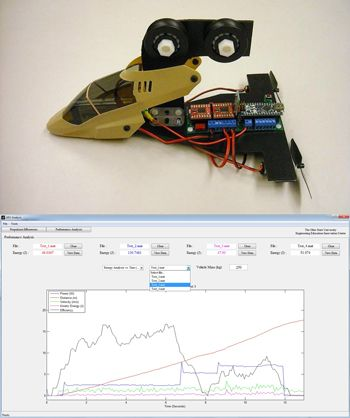Figure 4. Top: A monorail vehicle. Bottom: MATLAB interface used to plot power consumption, distance traveled, velocity, kinetic energy, and energy efficiency as a function of time.

In upper-level courses and in their final-year capstone projects, students build upon the skills they’ve developed in MATLAB throughout the First-Year Engineering program. For example, in ENGR 2167: Data Acquisition with MATLAB, students explore the use of data acquisition (DAQ) devices, including the USB-6009 and myDAQ from National Instruments. During one exercise, a ball is dropped through a vertical tube lined with break-beam sensors (Figure 5). Using a DAQ device in session mode with Data Acquisition Toolbox™, we collect data from the sensors at 20,000 samples per second. We use MATLAB to compute the ball’s velocity as it falls, and compare the result to the velocity of a ball dropping in a vacuum, calculated from Newton’s laws.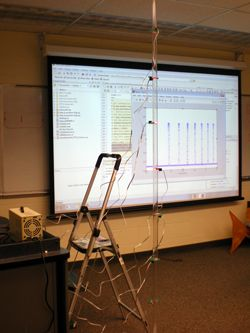Figure 5. DAQ setup for measuring the velocity of a dropped ball using MATLAB and Data Acquisition Toolbox.

## High-Fives and Other Positive Feedback

By the end of their first year, the students have a solid grasp of data analysis and programming with MATLAB and understand how vectors, indexing, loops, and conditionals work. The train project has led not only to high-fives in the lab, but also to very favorable course reviews from our students.

Since the adoption of the First-Year Engineering course sequence with MATLAB, a higher percentage of students are opting to continue in the engineering program. We believe that the increased retention rate is partly due to the students having experienced the satisfaction of facing and overcoming a genuine engineering challenge in their first year.